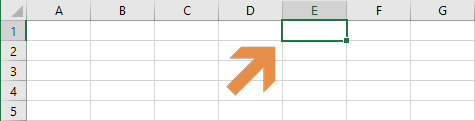In this guide, we're going to show you how to refer a range or a cell in Excel VBA.

## VBA Basics

Before diving into the code, let’s start with a cell or a range means in VBA. First, VBA is an object-based programming language, which means that the language refers each element as objects. Objects have properties that define them, and they can encapsulate other objects, or codes. Thus, a single cell, a range of cells, a worksheet, or Excel software as a whole is an object for VBA. You can think this as a hierarchical model.The image illustrates only a small portion of Excel objects in VBA. An Excel instance contains a Workbooks collection. A collection is a group of related objects. For example, if you open two workbooks, the Workbooks collection has two Workbook object. Each Workbook object has its own worksheets under a Worksheets collection. This structure applies to all.

VBA envelops all cell and cell-based references in an object called Range. In theory, when a particular object is referenced, you also need to specify its parents. In VBA syntax, a dot (.) operator is used to move through object hierarchy. For example, to access the Range object, the code should be:

`Application.Workbooks.Worksheets.Range`

However, this reference is ambiguous when it comes to specify which Excel workbook or worksheet you are referring to. In order to do this, you must supply the name or the index number (starting with 1) of the particular object you are referring. This approach is like in Excel formulas - the argument is given in parentheses. For example to refer the range object in the worksheet “Sheet1” of the workbook “Book1.xlsm”:

`Application.Workbooks("Book1.xlsm").Worksheets("Sheet1").Range`

or

`Application.Workbooks(1).Worksheets(1).Range`

You do not have to specify all parents for Range object every time. If your intention is to work on the active sheet of the active workbook, you can simply use Range.

## Range Object

The Range object allows you to refer a cell or cells just like in any Excel formula. For example,

```Range("A1") 'Refers the cell A1
Range("A2:D11") 'Refers the range of cells between A2:D11
Range("A3,B4:E6,F12") 'Refers all cells in the cells A3, F12 and the range B4:E6
Range("2:2") 'Entire 2nd row
Range("E:E") 'Entire column E
Range("Input") 'Named range "Input"```

## Using square brackets to refer a range or a cell

You can use square brackets ([]) instead of the “Range” keyword and double quotes (“). This approach as essentially a shortcut, and the outcome is the same.

```[A1] 'Refers the cell A1
[A2:D11] 'Refers the range of cells between A2:D11
[A3,B4:E6,F12] 'Refers all cells in the cells A3, F12 and the range B4:E6
[2:2] 'Entire 2nd row
[E:E] 'Entire column E
[Input] 'Named range "Input"
```

## Cells property to refer a range or a cell

Cells is the name of a property of both Worksheet and Range objects. It is neither a collection nor an object. Thus, there are no objects named Cell.

In other words, the Cells property returns a Range object as well. Each Cells property in Worksheet and Range objects work in its parent’s context only. This behavior might make more sense within examples.

### Syntax

The Cells property can be used in two ways:
With row and column index numbers:

`Cells(row number,column number) `

With index number of the cell:

`Cells(cell number) ‘refers the 23rd cell in the range`

### Examples

#### Worksheet.Cells

Cells property of a Worksheet object returns the cell in a specific location in the worksheet.
With row and column index numbers:

`Cells(3,2) 'refers the cell at third row and second column in the active sheet (B3)`
`Cells(5) 'refers the 5th cell in the range (E1)`The numbering of cells starts from 1, and increases from left to right and from top to bottom. This means that A1 is the first cell.

#### Range.Cells

On the other hand, the Cells property of a Range object returns the cell in specified location in the range.
With row and column index numbers:

`Range(&quot;C4:F9&quot;).Cells(3,2) 'refers the cell at third row and second column in the active range (D6)`
`Range(&quot;C4:F9&quot;).Cells(5) ‘refers the 5th cell in the range (C5)`## Active objects to refer a range or a cell

There are keywords in VBA to reference active(selected) objects when the code is running.

```ActiveCell 'Refers the selected cell
Selection 'Refers any selected object. This can be cells or a chart
ActiveSheet 'Refers the active worksheet
ActiveWorkbook 'Refers the active worksheet
```